# Lesson 14 Function Argument in JavaScript

In the last lesson, we have discussed functions and its purpose, and we have learned how we can declare the function.
In this lesson, we are going a little bit deeper into the function and will discuss in detail about how we can define a function with arguments.

## Function Arguments in JavaScript

Till now, we have used functions without Arguments, in the last lesson we have declared simple boostlog() function to print ("Hey, Welcome to Boostlog!") message.
But with arguments, we can add more functionality to our function statement to make our program more efficient.
So arguments are the built-in object of JavaScript.
It does not perform any action rather than passing values to functions and it acts as a local variable.
Arguments make our code more flexible and dynamic.

### Syntax

Here is the basic syntax which shows how we use arguments function:

``````function ourFunctionName(Argument) {
// Bunch of code to be executed
}
``````

The basic structure of the function is almost same but we have defined Arguments inside of parentheses.
We can use multiple arguments inside parentheses which we separate by commas.

### Example

So let’s try some simple example here, we are writing a JavaScript program to greet user by name, for example, if someone visits our site so we greet them by name like ("Hello Name, Welcome to Boostlog!")

### JavaScript Code

``````function greeting(username){
console.log("Hello "+ username +", Welcome to Boostlog!");
}
``````

You can notice in this code we have a function whose name is greeting and inside parentheses, and then we have defined a parameter ‘username’ and used that parameter in console.log message dialog.
Now we can call this function from anywhere.
You can notice that we still have not defined ‘username’ argument anywhere let suppose our username is “Alex”.
So when we call the function we will place “Alex” as the argument.
So we pass the value to function using arguments.
Here is the code below to call the function.

``````greeting(“Alex”);
``````

In this case, the username argument value Alex passed to function, so whenever we call username represent “Alex”.
Here is complete code below:

``````function greeting(username){
console.log("Hello "+ username +", Welcome to Boostlog!");
}
greeting("Alex");
``````

### Output

So when we run this code we will get the following output.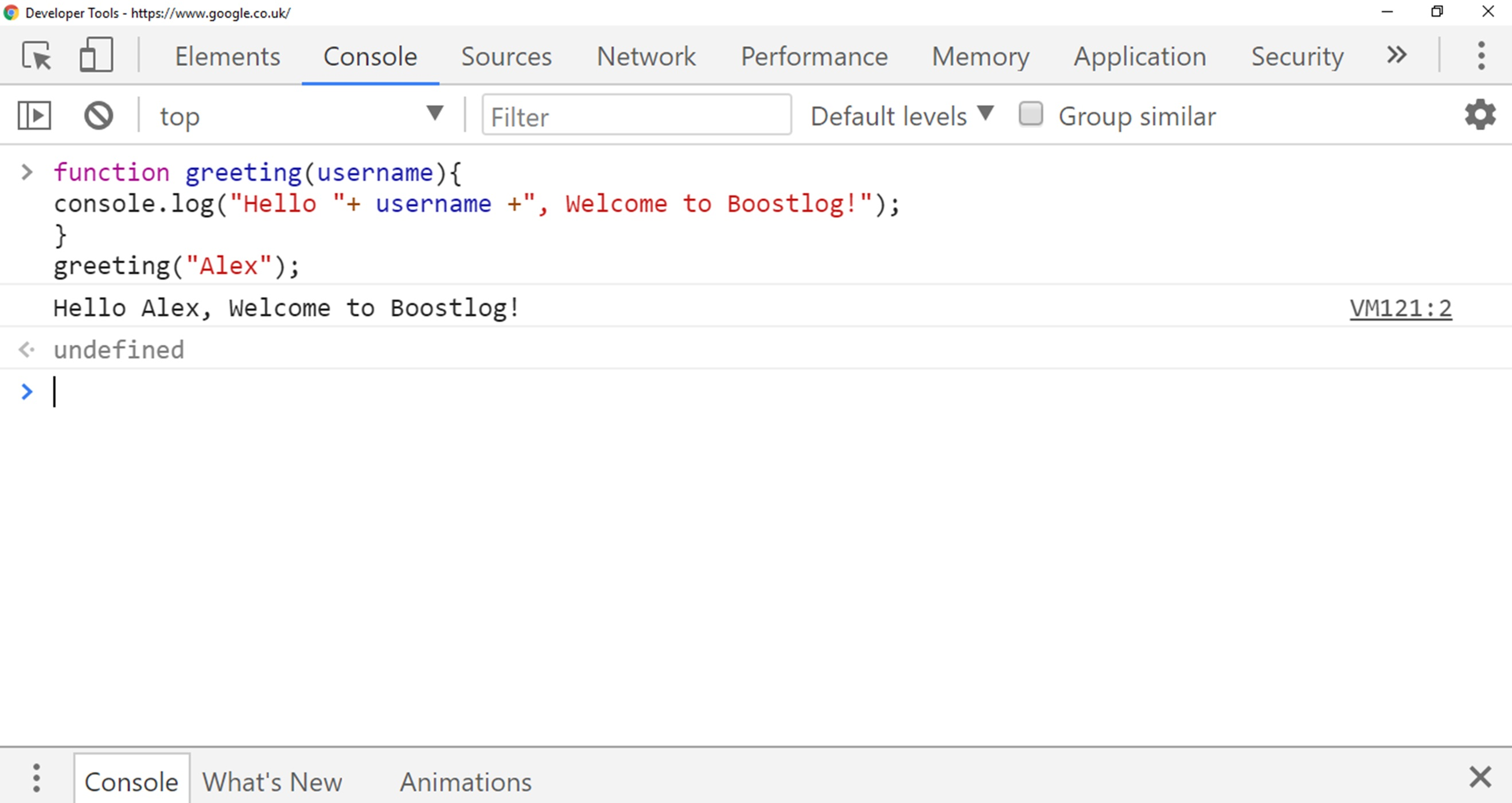You can notice in above output, whatever value we passed in Argument, function immediately that value and that makes function super powerful.

## Passing Multiple Arguments

In JavaScript we are not limited to pass a single argument, we can pass multiple arguments inside parentheses.
So here we are creating a function that has two arguments and the purpose of this function is to multiply arguments and then print them.

## JavaScript Code

``````function greeting(no1, no2){
console.log(no1*no2);
}
greeting(10, 20);
``````

In above code, we have used two arguments no1 and no2 and multiply the value of these two arguments in console.log and print the result, in this case, value 10 and 20 passed through no1 and no2 parameters.
Here is the output below: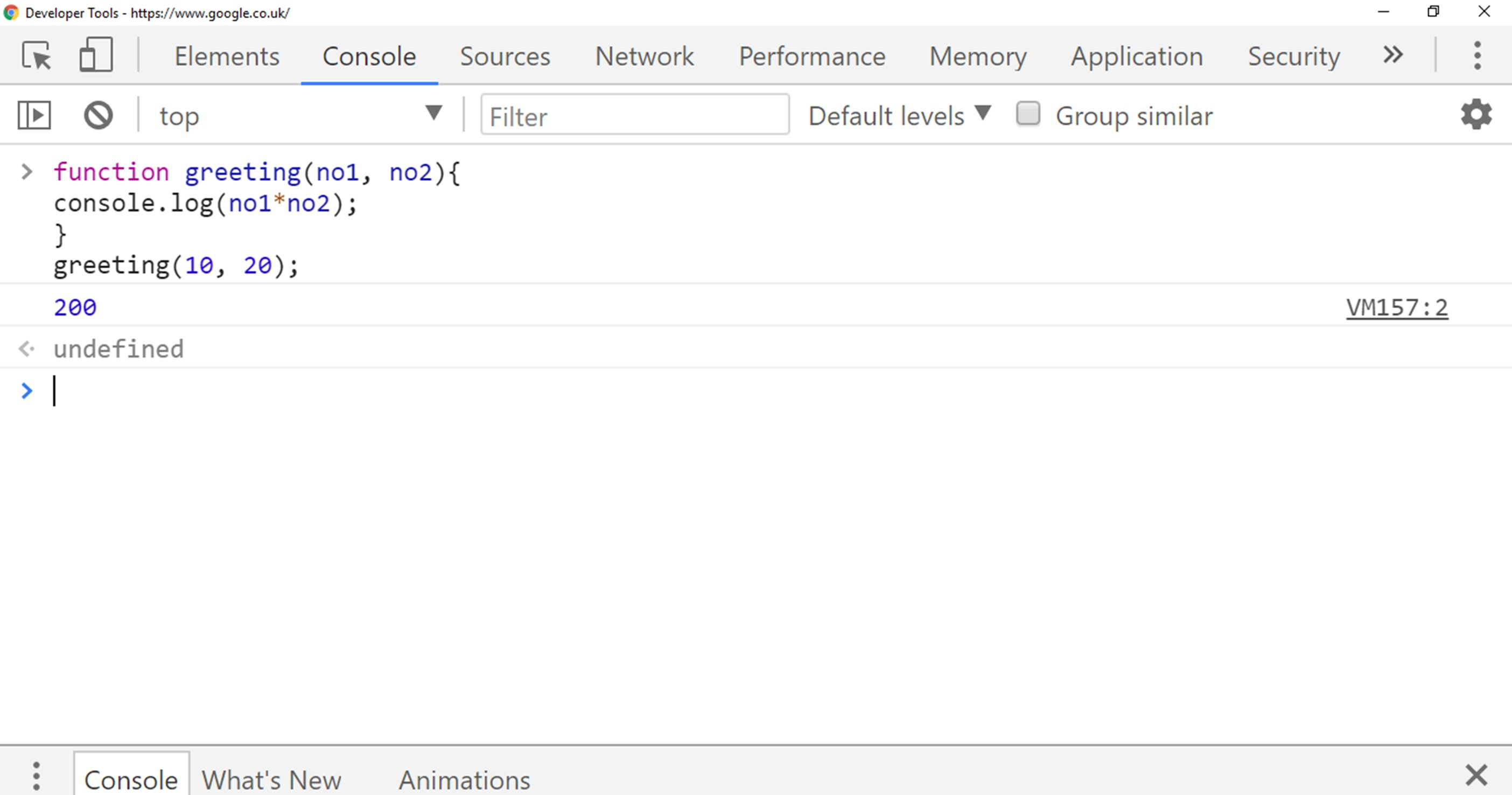## Example

So if we want to write a code whose function is to calculate the area of a rectangle and get the input length and width from the user.
So we usually multiply length and width to find the area of the rectangle, in JavaScript, it is much more similar to mathematics.

## JavaScript Code

``````function areaRectangle(length, width){
console.log(length*width);
}
var length = prompt("What's the Length of Rectangle");
var width = prompt("What's the Width of Rectangle");
areaRectangle(length, width);
``````

Here is the complete JavaScript code which get input length and width from user, multiply them and print answer.

### Output

Here is the output below, when we write above code in our console and press enter, then two prompt windows open and gets the input of length and width.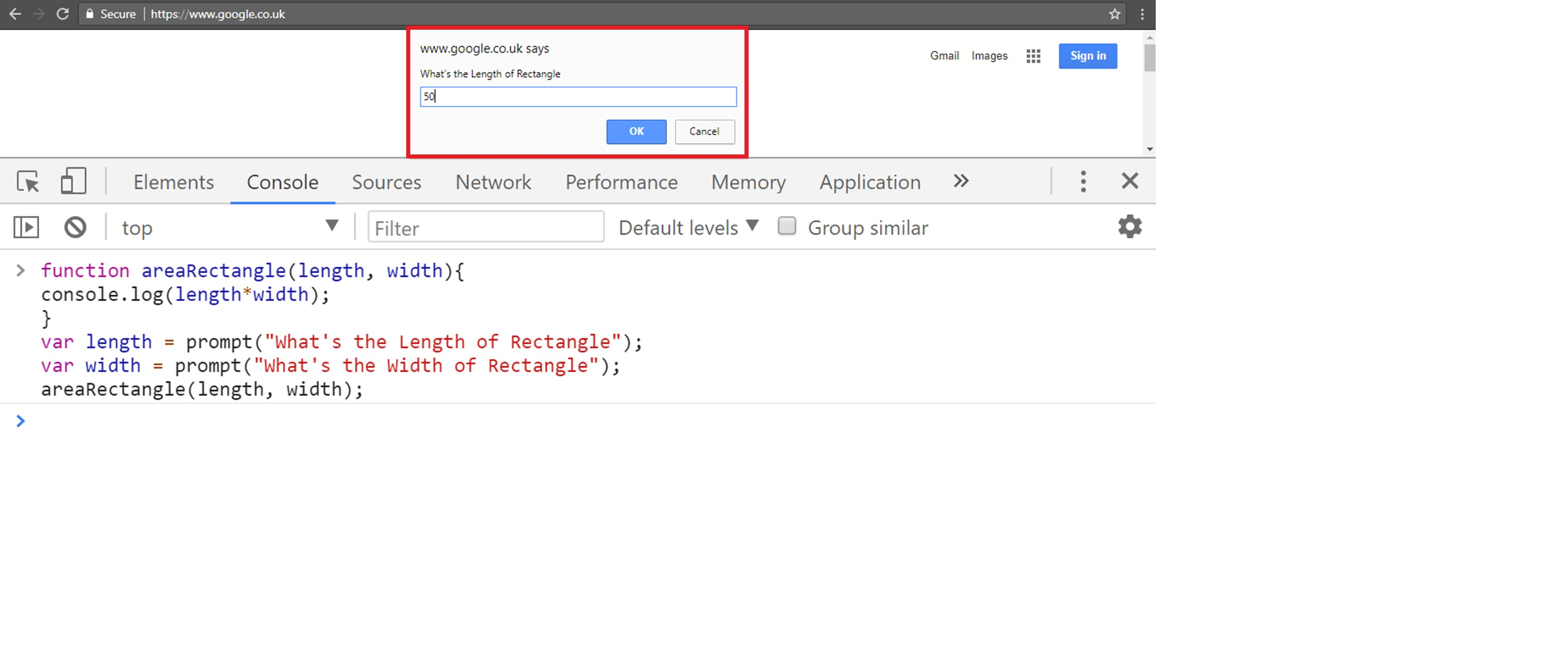You can see in above output, input box asking me to write the length of Rectangle.
When we wrote the length and press enter another input box opened and asked me to write width of the rectangle.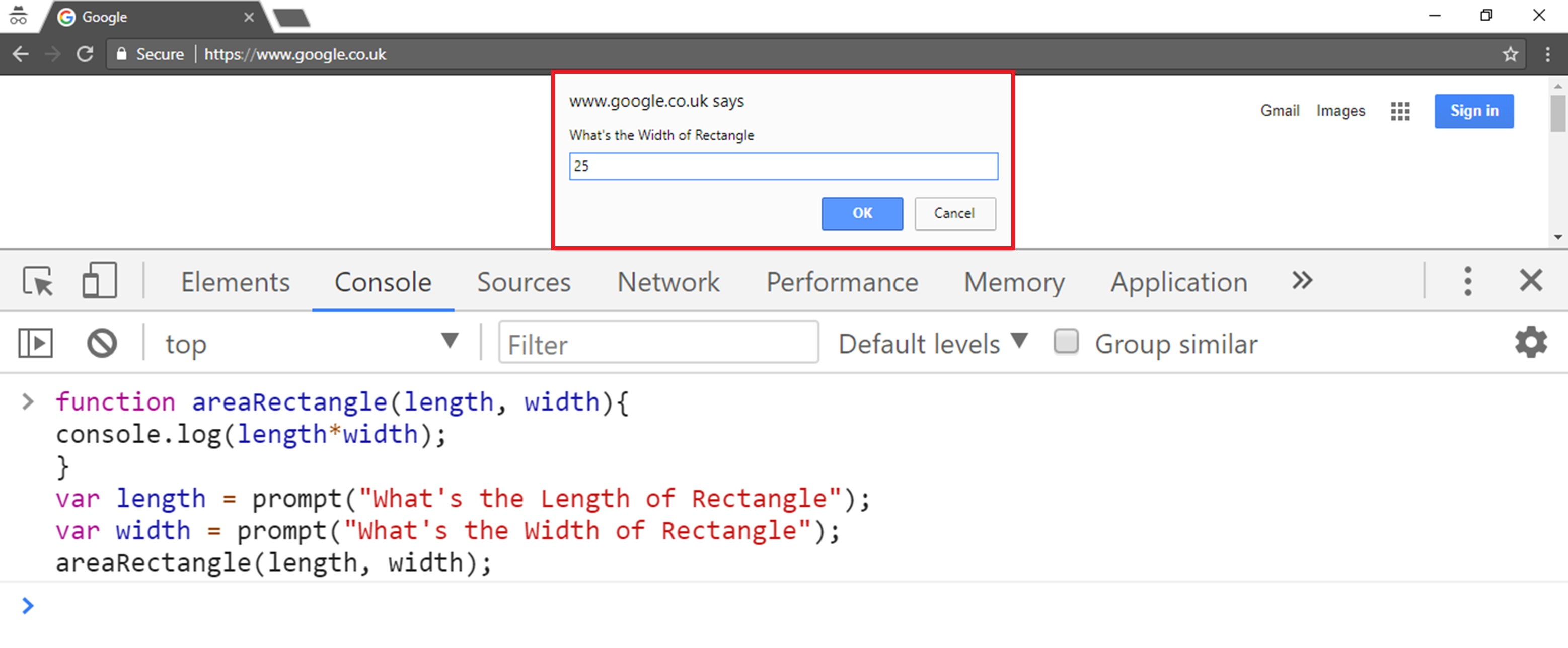So it printed the result which is 1250 when we enter length and width of the rectangle.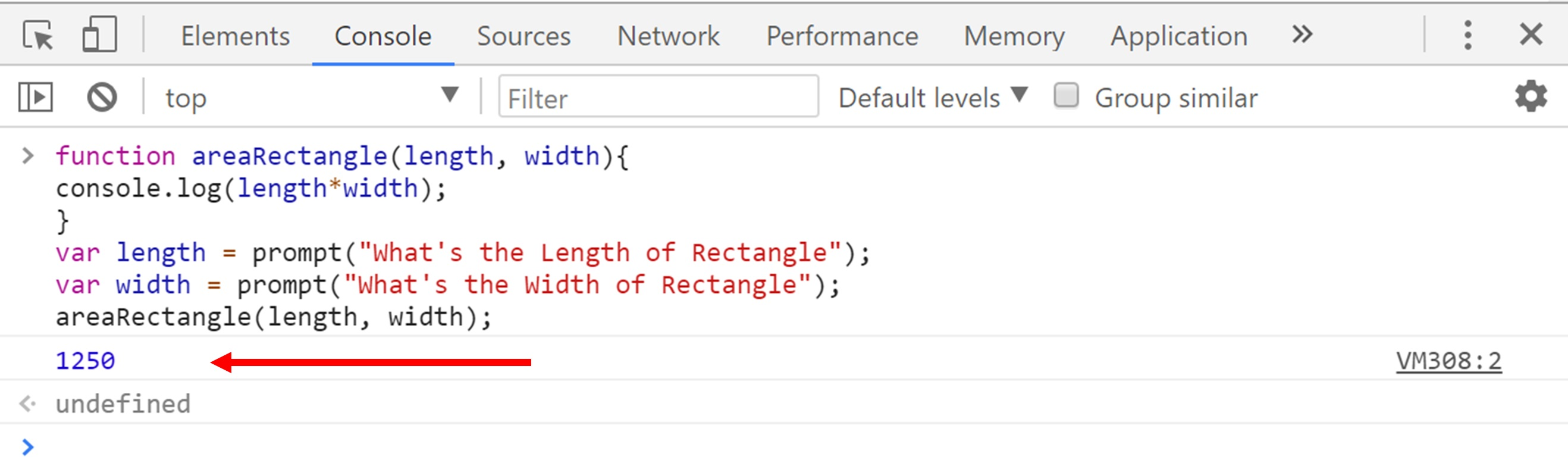## Conclusion

In this lesson, we have learned more about Arguments and discussed how we can pass arguments to the function and get inputs from users.
So JavaScript arguments are passed by values.
The function can only tell the values but it cannot tell the argument’s locations.